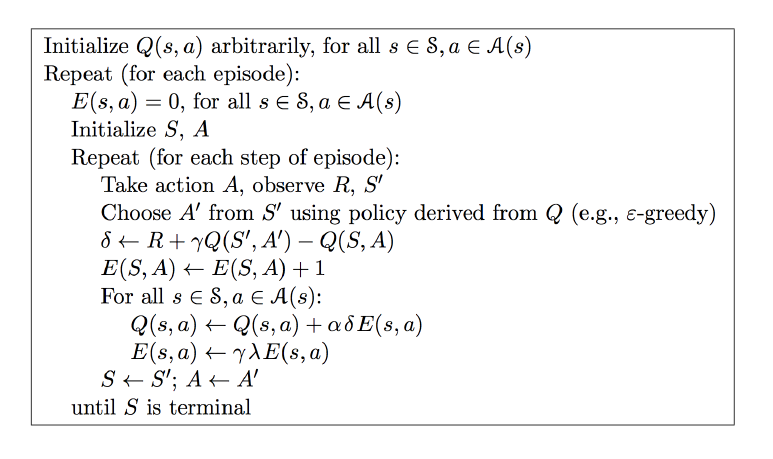强化学习（Q-Learning，Sarsa）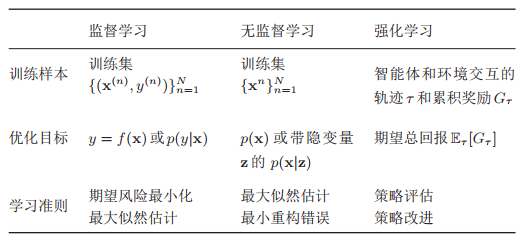Reinforcement Learning

• 没有监督标签。只会对当前状态进行奖惩和打分，其本身并不知道什么样的动作才是最好的。
• 评价有延迟。往往需要过一段时间，已经走了很多步后才知道当时选择是好是坏。有时候需要牺牲一部分当前利益以最优化未来奖励。
• 时间顺序性。每次行为都不是独立的数据，每一步都会影响下一步。目标也是如何优化一系列的动作序列以得到更好的结果。即应用场景往往是连续决策问题。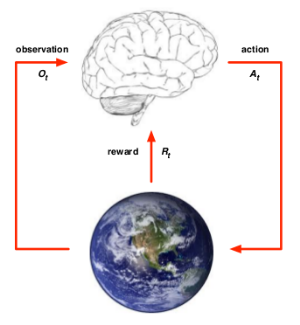Policy π：表示所采用的行动。它是从状态 S到行为A的一个映射，可以是确定性的，也可以是不确定性的。即动作将会根据策略 π(a|s) 这一概率分布来进行选择。

Value：状态价值函数，每种动作可能带来的后续奖励，用于评估策略。因为只有一个当前奖励 reward 是远远不够的，当前奖励大并不代表其未来的最终奖励一定是最大的，所以需要使用 Value期望函数来评价未来。
$v_π(s)=E_π(R_{t+1}+γR_{t+2}+γ^2R_{t+3}+...|S_t=s)$即是在s状态和策略π时，采取行动后的价值由接下来的时刻的R的期望组成，其中γ是奖励衰减因子，用于控制当前奖励和未来奖励的比重。越小越重视现在，由于环境不可全知，未来具有更多的不确定性。

Model：用来感知场景的变化。模型要解决两个问题：一是状态转化概率 $P^a_{ss′}$，即预测在s状态下，采取动作a,转到下一个状态s′的概率。另一个是预测可能获得的即时奖励$R_s^a$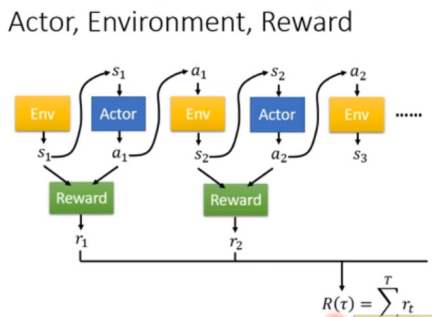$v_π(s)=E_π(R_{t+1}+γR_{t+2}+γ^2R_{t+3}+...|S_t=s)$$=E_π(R_{t+1}+γ(R_{t+2}+γR_{t+3}+...)|St=s)$$=E_π(R_{t+1}+γv_π(S_{t+1})|St=s)$

$q_{\pi}(s,a) = R_s^a + \gamma \sum\limits_{s' \in S}P_{ss'}^av_{\pi}(s')$

• 穷举法：把所有可能的路径和状况都试一遍计算，直接比较最后哪条路的总价值最大。
• 动态规划（DP）：将一个问题拆成几个子问题，分别求解这些子问题，反向推断出大问题的解。即要求最大的价值，那么根据递推关系，根据上一循环得到的价值函数来更新当前循环。但是它需要知道具体环境的转换模型，计算出实际的价值函数。相比穷举法，动态规划即考虑到了所有可能，但不完全走完。
• 蒙特卡洛（MC）：采样计算。即通过对采样的多个完整的回合（如玩多次游戏直到游戏结束），在回合结束后来完成参数的计算，求平均收获的期望并对状态对动作的重复出现进行计算，最后再进行更新。$G_t =R_{t+1} + \gamma R_{t+2} + \gamma^2R_{t+3}+... \gamma^{T-t-1}R_{T}$$N(S_t, A_t) = N(S_t, A_t) +1$状态价值函数和动作价值函数的更新就为：$V(S_t) = V(S_t) + \frac{1}{N(S_t)}(G_t - V(S_t) )$$Q(S_t, A_t) = Q(S_t, A_t) + \frac{1}{N(S_t, A_t)}(G_t - Q(S_t, A_t) )$
这种方法是价值函数q的无偏估计，但方差高（因为需要评价每次完成单次采样的结果，波动往往很大）。
• 时序差分（TD）：步步更新。不用知道全局，走一步看一步的做自身引导。即此时与下一时刻的价值函数差分（也可以理解是现实与预测值的差距）来近似代替蒙特卡洛中的完整价值。$G(t) = R_{t+1} + \gamma V(S_{t+1})$那么：$V(S_t) = V(S_t) + \alpha(G_t - V(S_t) )$$Q(S_t, A_t) = Q(S_t, A_t) +\alpha(G_t - Q(S_t, A_t) )$所以它是有偏估计，但是方差小，也便于计算，在实践中往往用的最多。

Q-Learning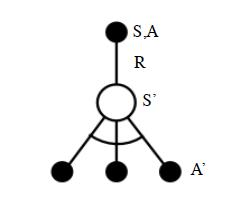Q-Learnig的思想就是，如上图从上到下，先基于当前状态S，使用ϵ−贪婪法按一定概率选择动作A，然后得到奖励R，并更新进入新状态S′，基于状态S′，直接使用贪婪法从所有的动作中选择最优的A′（即离线选择，不是用同样的ϵ−贪婪）。$Q(S,A) = Q(S,A) + \alpha(R+\gamma \max_aQ(S',a) - Q(S,A))$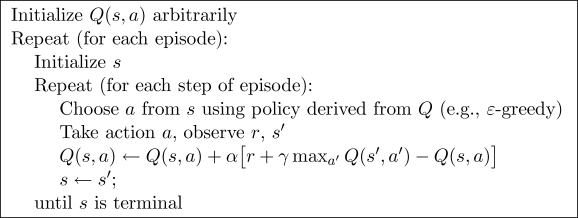Sarsa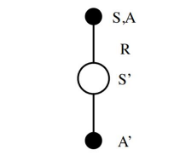Sarsa的思想和Q-Learning类似。如上图从上到下，先基于当前状态S，使用ϵ−贪婪法按一定概率选择动作A，然后得到奖励R，并更新进入新状态S′，基于状态S′，使用ϵ−贪婪法选择A′（即在线选择，仍然使用同样的ϵ−贪婪）。$Q(S,A) = Q(S,A) + \alpha(R+\gamma Q(S',A') - Q(S,A))$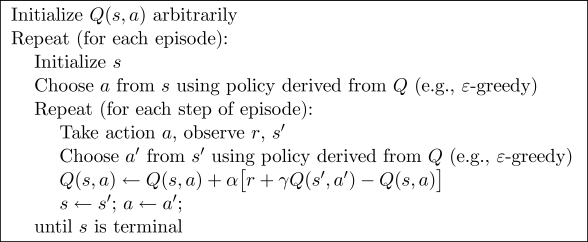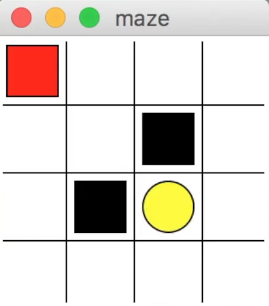#this
def update():
# 学习 100 回合
for episode in range(100):
# 初始化 state 的观测值
observation = env.reset() #env是搭建的游戏环境

while True:
env.render() # 刷新可视化环境

# 根据当前s 的观测值挑选 a，观测值是环境返回，这里返回的是小红的坐标
action = RL.choose_action(str(observation))

# 执行action, 得到s', r，和终止符done
observation_, reward, done = env.step(action)

# 从这个序列 (state, action, reward, state_) 中学习
RL.learn(str(observation), action, reward, str(observation_))

# 将下一个 state 的值传到下一次循环
observation = observation_

if done:
break

# 结束游戏并关闭窗口
print('game over')
env.destroy()

#RL
import numpy as np
import pandas as pd

class QLearningTable: #关于Q表
def __init__(self, actions, learning_rate=0.01, reward_decay=0.9, e_greedy=0.9):
self.actions = actions  #选动作，有上下左右
self.lr = learning_rate #学习率
self.gamma = reward_decay #奖励衰减
self.epsilon = e_greedy #贪婪系数
self.q_table = pd.DataFrame(columns=self.actions, dtype=np.float64) #初始Q表

def choose_action(self, observation):
self.check_state_exist(observation)#检测该状态是否存在，不存在就新建
if np.random.uniform() < self.epsilon: #随机数如果小于epsilon就选择最好的动作，如0.9的概率会选择最大
state_action = self.q_table.loc[observation, :]
# 针对同一个 state, 而不同动作的Q值却相同，采取随机选择
action = np.random.choice(state_action[state_action == np.max(state_action)].index)
else: #0.1的概率探索没有去过的地方
action = np.random.choice(self.actions)
return action

def learn(self, s, a, r, s_):#更新Q表
self.check_state_exist(s_)#检测该状态是否存在，不存在就新建
q_predict = self.q_table.loc[s, a] #得到s和动作a的Q表值，即旧值
if s_ != 'terminal': #如果游戏没有结束
#贪婪的得到最大Q值乘学习率，加上reward得到s'和a'，即新值
q_target = r + self.gamma * self.q_table.loc[s_, :].max()
else:
q_target = r  #如果是终点，没有后续动作，就直接是0
self.q_table.loc[s, a] += self.lr * (q_target - q_predict)  # 时序差分新旧更新Q表



Sarsa的代码基本一样，在Q表更新的时候q_target = r + self.gamma * self.q_table.loc[s_, a_] ，即它直接选用已经确定了的a’而不是直接贪婪选max。

Sarsa lambda